## SBT Prize at the end of chapter 3 (C3 Math 7 – Horizon) – Math Book

SBT Prize at the end of chapter 3 (C3 Math 7 – Horizon)
==========

### Solve problem 1 page 64 SBT Math 7 Creative horizon episode 1 – CTST

Fill in the dot. A rectangular box (cube) has:

……. edge; ……. face; ……. top; …………. diagonal line; each vertex has ……. corner.

## Detailed instructions for solving Lesson 1

Solution method

Use properties of sides, faces, vertices, diagonals, corners of a rectangular box

Detailed explanation

A rectangular box (cube) has:

twelfth edge; 6 face; 8 top; 4 diagonal line; each vertex has 3 corner.

–>

— *****

### Solution 2 page 64 SBT Math 7 Creative horizon episode 1 – CTST

Let ABCD.EFGH rectangular box.

a) Name the faces containing the edge EF.

b) What is the edge of GH?

c) Draw a diagonal starting from vertices E, G.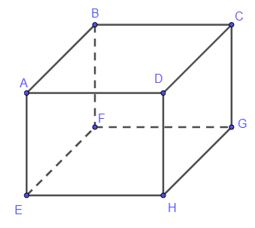## Detailed instructions for solving Lesson 2

Solution method

Using properties of rectangles

Detailed explanation

Observing rectangular box ABCD.EFGH, we have:

a) The faces containing the edge EF are: surface ABFE, face EFGH.

b) The sides equal to the side GH are: EF, CD, AB (we can write GH = EF = CD = AB).

c) The diagonal from vertex E is EC, the diagonal from vertex G is GA.

–>

— *****

### Solve problem 3 pages 64 SBT Math 7 Creative horizon episode 1 – CTST

Nam intends to use a cut iron bar to make a 30 cm cube frame. Is a 3.5 m long iron rod enough to make a frame?

## Detailed instructions for solving Lesson 3

Solution method

Use the property of a cube with 12 equal sides to calculate the total length of the sides and then compare it with a 3.5m long iron bar.

Detailed explanation

A cube has 12 equal sides, 30 cm each.

Hence the lengths of all sides are: 12 . 30 = 360 (cm).

Change 360 ​​cm = 3.6 m.

That 3.5 m < 3.6 m.

So the iron rod is not long enough to make the frame.

–>

— *****

### Solve problems 4 pages 64 SBT Math 7 Creative horizon episode 1 – CTST

One needs to make a rectangular box with dimensions of 2cm, 3cm and 5cm.

a) Show two ways to cut the cardboard to make the box above.

b) Calculate the area of ​​the cardboard after cutting in each case.

## Detailed instructions for solving Lesson 4

Solution method

Method 1: On the card, draw 2 rectangles with the dimensions as shown below, then fold along the dashed lines to get a satisfying rectangular box.

Method 2: On the card, draw 2 rectangles with the dimensions as shown below, then fold along the dashed lines to get a satisfying rectangular box.

Detailed explanation

a)

Method 1: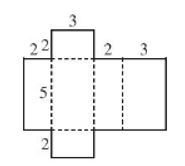Method 2: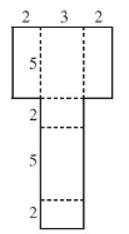b) The area of ​​the cardboard after cutting in the above two ways is equal to the total area of ​​the rectangular box, so the area of ​​the cardboard after cutting in Method 1 is equal to the area of ​​the card after cutting in Method 2 and is: S = 2 . (2 . 3 + 2 . 5 + 3 . 5) = 62 (cm2)

–>

— *****

### Solve problems 5 pages 64 SBT Math 7 Creative horizon episode 1 – CTST

From a rectangular card, show two ways of cutting and folding to form a vertical prism whose base is an equilateral triangle of side 3 cm and height 2 cm.

## Detailed instructions for solving Lesson 5

Solution method

Method 1: On the card, we draw two equilateral triangles with side 3 cm and 3 equal rectangles with the same dimensions as shown in the figure and then fold along the dashed lines, we get a vertical prism satisfying the requirements.

Method 2: On the card, we draw two equilateral triangles with side 3 cm and 3 equal rectangles with the same dimensions as shown in the figure and then fold along the dashed lines, we get a vertical prism satisfying the requirements.

Detailed explanation

Method 1: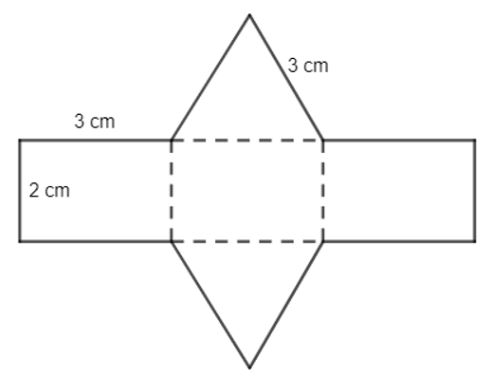Method 2: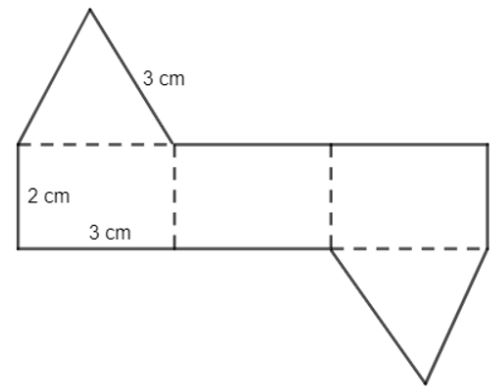–>

— *****

### Solve problem 6 pages 64 SBT Math 7 Creative horizon episode 1 – CTST

Which of the four cards below cannot be folded into a triangular prism?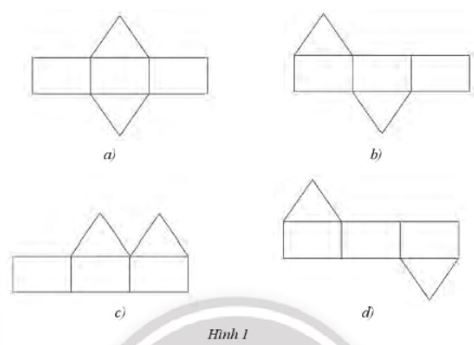## Detailed instructions for solving Lesson 6

Solution method

Use properties of triangular prisms, to see which shapes satisfy the requirement

Detailed explanation

– According to Lesson 5we see that the cardboard in Figure 1a) and Figure 1d) can be folded into a triangular prism.

– We fold the cover of Figure 1b) along the dashed lines as shown below, we get a triangular prism.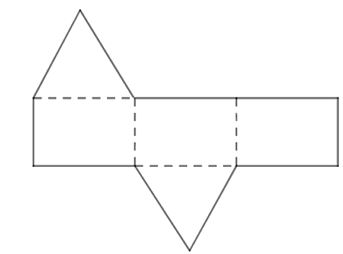– In the cover of Figure 1c), the two triangles are on the same side, so it cannot be folded to form 2 bases of the triangular prism (because the two bases of the triangular prism must be parallel to each other) .

So the sheet of Figure 1c) cannot be folded into a triangular prism.

–>

— *****

### Solution 7 page 65 SBT Math 7 Creative horizon episode 1 – CTST

A metal block in the shape of a prism stands at the base of a right triangle with the dimensions of the two sides, the right angle being 3 cm, 4 cm, and the hypotenuse 5 cm. A prism-shaped hole is drilled at the base of a right triangle with two right-angled sides measuring 2 cm, 1.5 cm, hypotenuse 2.5 cm (Figure 2). Calculate the volume of the metal block (excluding the hole).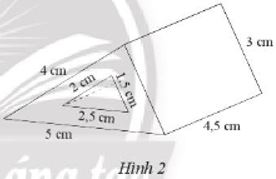## Detailed instructions for solving Lesson 7

Solution method

We calculate the volume of the whole metal block when the hole is included and then subtract the volume of the hole to get the volume of the metal block excluding the hole.

Detailed explanation

+ The metal block has the shape of a triangular prism with the base is a right triangle with the dimensions of the two sides, the right angles are 3 cm, 4 cm and the height of the prism is h = 4.5 cm.

Then, the volume of the metal block (including the hole) is:

V = WILLD . h = (\(\dfrac{1}{2}\). 3 . 4) . 4.5 = 27 (cm3).

+ The hole has the shape of a triangular vertical prism with a right triangle base with two sides measuring 2 cm, 1.5 cm, and the height of the prism is h = 4.5 cm (equal to the height of the prism). of a metal prism).

Then the volume of the hole is:

V = WILLdn . h = (\(\dfrac{1}{2}\). 2 . 1.5) . 4.5 = 6.75 (cm3).

So the volume of the metal block (excluding the hole) is: 27 – 6.75 = 20.25 (cm .)3).

–>

— *****

### Solve problem 8 page 65 SBT Math 7 Creative horizon episode 1 – CTST

Fired solid brick (Figure 3) is a type of brick made of clay and fired monolithically, without voids. Due to the solid structure, the brick block is quite solid, less water-absorbent, ensuring the structure of the work. Uncle Ba wants to make 500 bricks like that, how many cubic meters of clay are needed? Know the size of each brick is 205 mm, 95 mm, 55 mm, and the expansion is negligible.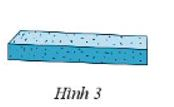## Detailed instructions for solving Lesson 8

Solution method

Calculate the volume of 1 brick and then calculate the volume of the clay block that makes 500 bricks

Detailed explanation

The volume of a fired brick is: 205 . 95 . 55 = 1 071 125 (mm3).

The volume of the clay block needed to make 500 fired bricks is:

500 . 1 071 125 = 535 562 500 (mm3).

Change 535 562 500 mm3 = 0.5355625 m3 0.54 m3.

So it takes about 0.54 cubic meters of clay to make 500 bricks.

–>

— *****